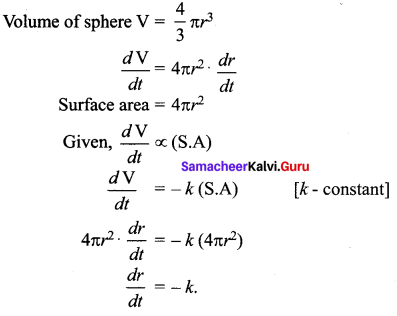# Samacheer Kalvi 12th Maths Solutions Chapter 10 Ordinary Differential Equations Ex 10.2

## Tamilnadu Samacheer Kalvi 12th Maths Solutions Chapter 10 Ordinary Differential Equations Ex 10.2

Question 1.
Express each of the following physical statements in the form of differential equation.
(i) Radium decays at a rate proportional to the amount Q present.
Solution:
Radium decays at a rate proportional to the amount Q present.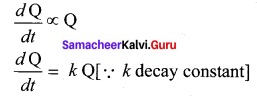(ii) The population P of a city increases at a rate proportional to the product of population and to the difference between 5,00,000 and the population.
Solution:
Rate of change of P with respect to ‘t’ is $$\frac{d \mathrm{P}}{d t}$$
Product of population and the difference between 50,000 and the population is P (50,000 – P)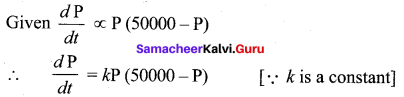(iii) For a certain substance, the rate of change of vapor pressure P with respect to temperature T is proportional to the vapor pressure and inversely proportional to the square of the temperature.
Solution: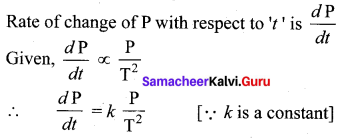(iv) A saving amount pays 8% interest per year, compounded continuously. In addition, the income from another investment is credited to the amount continuously at the rate of ₹ 400 per year.
Solution:
Let ‘x’ be the amount invested.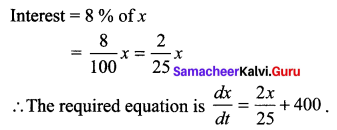Question 2.
Assume that a spherical rain drop evaporates at a rate proportional to its surface area. Form a differential equation involving the rate of change of the radius of the rain drop.
Solution: